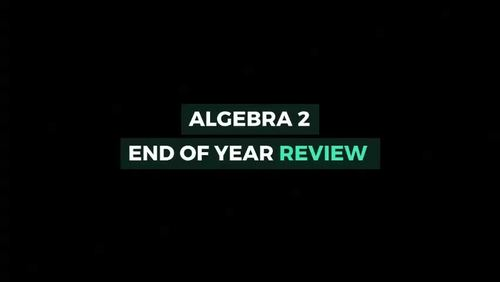End of Year Algebra 2 Review or PreCalculus summer packetSubject
Resource Type
Product Rating
4.0
5 Ratings
File Type

Compressed Zip File

8 MB|36+
Share
Also included in:
1. This bundle includes my ENTIRE library of Algebra 2 notes, activities and projects PLUS all future Algebra 2 resources. Interactive notebook foldables and notes, math detectives, coded messages, paper chains, task cards, projects and more fill this 500+ page bundle. Current bundle of resources val
\$420.95
\$294.66
Save \$126.29
Product Description

Use as an end of year review for Algebra 2 final exam OR as a summer math for students who have just completed Algebra 2 to maintain their knowledge in the summer OR for back to school in Pre-Calculus with a quick review! It also works well as a no-prep emergency sub packet! Examples, explanations and practice problems included for each topic. You can download a FREE sample of pages here.

This resource includes review noes for the following topics plus 188 practice problems (scroll down for a full listing of topics and subtopics):

• Slope
• Linear Equations
• Systems of Equations
• Polynomials
• Logarithms and Exponents
• Conic Sections
• Rational Functions
• Sequences and Series
• Trigonometry (introductory)

This purchase is for one teacher only. This resource is not to be shared with colleagues or used by an entire grade level, school, or district without purchasing the proper number of licenses. If you are interested in a site license, please contact me for a quote at docrunning@kulikuli.net. This resource may not be uploaded to the internet in any form, including classroom/personal websites or network drives.

LINEAR EQUATIONS

• Slope
• Linear equations
• Parallel and perpendicular lines
• Write the equation of a line
• Absolute Value functions

SYSTEMS OF EQUATIONS

• Substitution
• Elimination
• Graphing

• Vertex and standard form
• Factoring
• Solving
• Complex numbers

POLYNOMIALS

• Operations with polynomials
• Long and synthetic division
• Factoring polynomials

• Laws of Exponents
• Scientific Notation
• Operations with functions
• Combination of Functions

EXPONENTIAL AND LOGARITHMIC FUNCTIONS

• Graphing exponential functions
• Solving exponential functions with and without logarithms
• Properties of logarithms
• Solving logarithmic equations

• Inverse and Joint Variations
• Operations with rational expressions
• Solving rational equations

CONIC SECTIONS

• Parabola, circle, ellipse, hyperbola
• Applications of conic sections

SEQUENCES AND SERIES

• Arithmetic Sequences
• Geometric Sequences
• Arithmetic Series
• Geometric Series
• Infinite Geometric Series

TRIGONOMETRY

• Trigonometric functions
• Law of Sines
• Law of Cosines
• Area of triangle using trigonometry (2 methods)
Total Pages
36+